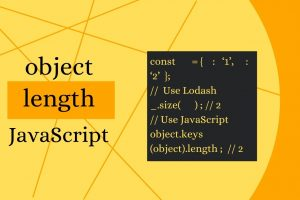# How to Get Length of JavaScript Object

JavaScript objects allow you to store diverse data in compact manner as key-value pairs. JS objects make it easy to access elements using their keys. Sometimes you may need to calculate the length of JavaScript object. In this article, we will learn how to get length of JavaScript object.

## How to Get Length of JavaScript Object

Let us say you have the following JS object.

`var obj = {1:'a',2:'b',3:'c', 4:null};`

Here is a simple code to get the length of the above object.

```var size = 0, key;
for (key in obj) {
if (obj.hasOwnProperty(key)) size++;
}
console.log(size);//contains the length of object as 4```

In the above code, we just iterate through the object, check if each item has key as its property, increments the size by 1. If the key is not a direct property of the object, then it is not included in the length calculation. Please note, even if the key is null or undefined but present as one of the keys, it is counted in length calculation. For example, in the above example, the key ‘4’ is counted even through its value is null.

You can use the above code to create a prototype called ‘size’ that is available for each JS object.

```Object.size = function(obj) {
var size = 0, key;
for (key in obj) {
if (obj.hasOwnProperty(key)) size++;
}
return size;
};

// Get the size of an object
const myObj = {1:'a',2:'b',3:'c', 4:null}
var size = Object.size(myObj); // size=4```

For modern browsers, you can also use length property available for Object.keys().

`var size = Object.keys(myObj).length;`

The above method works for all properties except symbolic properties. For this purpose, you need to use getOwnPropertySymbols() function. Here are a couple of examples.

```var person = {
[Symbol('name')]: 'John',
[Symbol('age')]: 33,
"occupation": "Programmer"
};

const propOwn = Object.getOwnPropertyNames(person);
console.log(propOwn.length); // 1

let propSymb = Object.getOwnPropertySymbols(person);
console.log(propSymb.length); // 2```

In this article, we have learnt how to get length of JavaScript object.